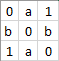# Improving precision score by applying weight matrix to cross_entropy loss function?

Hello fellow Pytorchers!

I am working on a CNN to make directional predictions of a market based on a visual representation of the recent market state. The model can make three classifications, down, zero and up. I use the torch.nn.functional.cross_entropy function to determine the loss, which does its job.

However, for practical purposes I want to specifically optimize the precision score of the up and down predictions. To do this, I need to penalize up-predictions that are actually down harder than misclassified zero-predictions for example. I have an evenly distributed set of labels and I know I can apply loss_weight to force more zero-predictions, which slightly improves the precision scores for the up and down predictions.

However, I am looking to apply a weight matrix instead of a vector to more specifically dictate loss, looking to further improve precision. Example of such a matrix would be the following:,
where 0<a<b<1

Is there any built-in functionality for this in Pytorch, or does this require me to do tinkering ‘under the hood’?

Cheers!

I’m not sure how your actual prediction look like, but assuming they would have the same shape as your weight matrix, you could simply multiply both before calling `loss.backward()`.

Let me know, if that would work!

Thanks for the reply!

I will clarify a little. The `output_logits` in the code below are `torch.Size([128, 3])`, a vector of 3 logit probabilities for every observation. My adjusted loss weights `loss_mod` is a `3*3` matrix.

I think I need the loss function to use this matrix along with the input and target. If I correctly understand how optimizing works, the direction in which the parameters are changed depends on the derivative of the loss function, right?

Looking at it more closely, I am wondering how `loss.backward()` and `optimizer.step()` are actually connected…

``````        loss_function = functional.cross_entropy
optimizer = torch.optim.Adam(model.parameters(), lr=learning_rate,)
#
#
for images_batch, labels_batch in train_data_loader:
images_batch = images_batch.to(device)
labels_batch = labels_batch.to(device)
optimizer.zero_grad()
output_logits = model(images_batch)
loss_mod = np.array([[0, 1/3, 1], [2/3, 0, 2/3], [1, 1/3, 0]])
loss = loss_function(input=output_logits, target=labels_batch)

loss.backward()
optimizer.step()

``````

Greatly appreciated looking at this!

Cheers!